##### The Japanese Bonsai specialist
Direct order Contact Help / Services Newsletter# Stone lantern yunoki 180 cm

› Lanterns, basins and statues › Stone lantern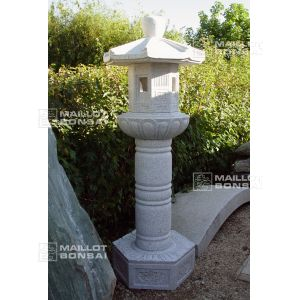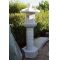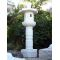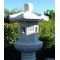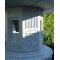ref. : 7427785,00

voluminous/heavy item extra shipping of 85,00

Available quantity : 1Order

###### Description

Height 180 cm. Ø 70 cm. Weight about 450 kilograms, delivered in 6 pieces to be easily assembled at home. A yunoki style lantern carved from solid granite, whose roof fans out gracefully and which contains a light box featuring square windows, fluting and textured detailing. The wide, skilfully carved lotus leaf centre table is supported by a spindle-like column and hexagonal base.

#lantern 5.7 #stone 4.5 #yunoki 3.6 #carved 2.6 #gracefully 2 #hexagonal 1.9 #skilfully 1.9 #supported 1.9 #delivered 1.9 #detailing 1.9

Formule
(( ROUND((CHAR_LENGTH(b.article_nom)-CHAR_LENGTH(REPLACE(b.article_nom, 'lantern', '')))/LENGTH('lantern')) + ROUND((CHAR_LENGTH(b.article_description)-CHAR_LENGTH(REPLACE(b.article_description, 'lantern', '')))/LENGTH('lantern')) ) * 3.7) + (( ROUND((CHAR_LENGTH(b.article_nom)-CHAR_LENGTH(REPLACE(b.article_nom, 'yunoki', '')))/LENGTH('yunoki')) + ROUND((CHAR_LENGTH(b.article_description)-CHAR_LENGTH(REPLACE(b.article_description, 'yunoki', '')))/LENGTH('yunoki')) ) * 3.6) + (( ROUND((CHAR_LENGTH(b.article_nom)-CHAR_LENGTH(REPLACE(b.article_nom, 'carved', '')))/LENGTH('carved')) + ROUND((CHAR_LENGTH(b.article_description)-CHAR_LENGTH(REPLACE(b.article_description, 'carved', '')))/LENGTH('carved')) ) * 2.6) + (( ROUND((CHAR_LENGTH(b.article_nom)-CHAR_LENGTH(REPLACE(b.article_nom, 'stone', '')))/LENGTH('stone')) + ROUND((CHAR_LENGTH(b.article_description)-CHAR_LENGTH(REPLACE(b.article_description, 'stone', '')))/LENGTH('stone')) ) * 2.5) + (( ROUND((CHAR_LENGTH(b.article_nom)-CHAR_LENGTH(REPLACE(b.article_nom, 'gracefully', '')))/LENGTH('gracefully')) + ROUND((CHAR_LENGTH(b.article_description)-CHAR_LENGTH(REPLACE(b.article_description, 'gracefully', '')))/LENGTH('gracefully')) ) * 2) + (( ROUND((CHAR_LENGTH(b.article_nom)-CHAR_LENGTH(REPLACE(b.article_nom, 'hexagonal', '')))/LENGTH('hexagonal')) + ROUND((CHAR_LENGTH(b.article_description)-CHAR_LENGTH(REPLACE(b.article_description, 'hexagonal', '')))/LENGTH('hexagonal')) ) * 1.9) + (( ROUND((CHAR_LENGTH(b.article_nom)-CHAR_LENGTH(REPLACE(b.article_nom, 'skilfully', '')))/LENGTH('skilfully')) + ROUND((CHAR_LENGTH(b.article_description)-CHAR_LENGTH(REPLACE(b.article_description, 'skilfully', '')))/LENGTH('skilfully')) ) * 1.9) + (( ROUND((CHAR_LENGTH(b.article_nom)-CHAR_LENGTH(REPLACE(b.article_nom, 'supported', '')))/LENGTH('supported')) + ROUND((CHAR_LENGTH(b.article_description)-CHAR_LENGTH(REPLACE(b.article_description, 'supported', '')))/LENGTH('supported')) ) * 1.9) + (( ROUND((CHAR_LENGTH(b.article_nom)-CHAR_LENGTH(REPLACE(b.article_nom, 'delivered', '')))/LENGTH('delivered')) + ROUND((CHAR_LENGTH(b.article_description)-CHAR_LENGTH(REPLACE(b.article_description, 'delivered', '')))/LENGTH('delivered')) ) * 1.9) + (( ROUND((CHAR_LENGTH(b.article_nom)-CHAR_LENGTH(REPLACE(b.article_nom, 'detailing', '')))/LENGTH('detailing')) + ROUND((CHAR_LENGTH(b.article_description)-CHAR_LENGTH(REPLACE(b.article_description, 'detailing', '')))/LENGTH('detailing')) ) * 1.9)

## Secure payment## Delivery

Our logistic partners :04 74 55 23 48
Pépinière MAILLOT-BONSAÏ
Le Bois Frazy
01990 RELEVANT - FRANCE
on appointment